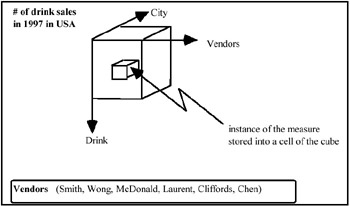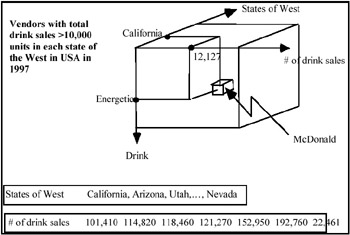# CHARACTERIZATION OF OLAP OPERATORS ON HIERARCHIES

Recently different authors proposed a set of OLAP operators, which are defined on the data cube and which produce a new cube as output (see Codd, Codd, & Salley, 1993; Agrawal, Gupta, & Sarawagi, 1997; OLAP Council, 1997; Cabibbo & Torlone, 1998). In this section, we discuss the operators involved in manipulating dimensions with hierarchies in order to introduce some important modifications and specializations.

### The Roll-up Operator

As mentioned above, this operator decreases the detail of the measure, aggregating it along the dimension hierarchy. A problem arises when a variable relative to a level of the hierarchy is not complete (i.e., in the case of a partial classification hierarchy).

In the following we consider what happens when this operator is applied to a total classification hierarchy and then to a partial classification hierarchy.

Example 6: Let us consider the data cube represented in Example 2. In Figure 11, its "multidimensional" view is illustrated.Figure 11: A Multidimensional View of the Drink Sales Data Cube

Let us suppose to formulate a query defined as below:

"Select Vendors for which the total Sales is >10000 units in each State in the West"

This query is solved in the following way:

Roll-up from City to Region, Select Region = West, Drill-down from Region to State, Push Vendors, Pull # of Drink sales, Dice # of Drink sales "10000."

Note that in some cells of the resulting cube (see Figure 12), null values can appear. This demonstrates that some instances of "Vendors" are not defined.Figure 12: The result of the query

Let us suppose, now, that the classification hierarchy relative to "Region State City" has, as domains, the cities of California, but with only the instances "San Francisco, San Jose, San Diego." This is a subset of the primitive domain of City, in which all the cities of California (San Francisco, San Jose, San Diego, Fresco, Los Angeles, etc.) are stored.

In particular, when the roll-up operator from City to State is applied, no information about the non-completeness of the City domain relative to California is stored. This means that for California, the number of vendors for which the total drinks sold in 1990 is >10,000 units refers only to the cities of San Francisco, San Jose, and San Diego, and not to all the cities in California. Therefore, since this information is not specified anywhere, the answer for this state is wrong.

A solution to this is to save the information about the domain values that cause the non-completeness of the hierarchy. This can be achieved in two different ways. The first is to add a Note (the clause where <variable name> is-a subset of the primitive domain) to the title of the cube. In the case of Figure 8, the title becomes "Vendors with total drink sales >10,000 units in each state in the West in 1997 in USA, where city of California is-a subset of the primitive domain." The second is to add the same Note to each variable of the hierarchy whose level is higher compared to that of the variable with the incomplete domain. In the same figure, we have to add the clause where city of California is-a subset of the primitive domain to the variables State, Region, and Country.

### The Slice Operator

As mentioned above, the slice operator reduces the dimensions (or cardinality) of a cube by eliminating one dimension through its multidimensional space. This fact is not always true because, if we delete a dimension whose domain is a subset of the primitive domain, we lose information and the resulting cube of this operation contains incorrect data.

Before discussing this situation, we need to introduce the implicit dimension definition.

Definition: We call any dimension of a data cube which has only one instance in its definition domain an implicit dimension. This instance can be one value or multi-valued.

Example 7: Let us consider the cube in Figure 6, where the primitive domain of the dimension Year assumes the values <1990, 1991, 1992, 1993, 1994, 1995, 1996, 1997, 1998>. This means that they are all the possible values which this dimension can assume in the database. Instead, the value domain of Year in the considered cube is <97, 98>.

Let us suppose that the following query is carried out:
"Give me the drink sales in all Cities by Class and Vendor"
It is solved in the following way:Slice Year

In this case if the slice operator deletes the dimension Year, the result seems to refer to the whole primitive domain of Year. This means that we lose the exact information on the real period to which the result should refer. To overcome this mistake, a specialization of the slice operator, called Implicit Slice (or I-Slice) was introduced (see Chapter 2). We remember its definition below.Definition The I-Slice operator removes the dimension on which it is applied, transforming it into an implicit dimension. The only value of the implicit dimension domain is all the values which formed the domain of the removed dimension.

Similarly to the solution proposed for the roll-up operator, the same Note is added to the title of the cube.

In accordance with this definition, the above query is now solved in the following way:I-Slice Year

The result is a cube with the same dimensions as the primary one, where the title becomes "Drink sales by Class, Vendor, and Year where Year is a subset of the primitive domain." For the symmetric reasoning of terminology, we use the term Total Slice (or T-Slice) for the well-known slice operator.

If, instead, the Year domain in the cube under consideration coincided with its primitive domain, the previous query would be solved in the following way:T-Slice Year

The cardinality of the resulting cube is now decreased by one dimension, since, by removing Year, no information is lost.Multidimensional Databases: Problems and Solutions
ISBN: 1591400538
EAN: 2147483647
Year: 2003
Pages: 150